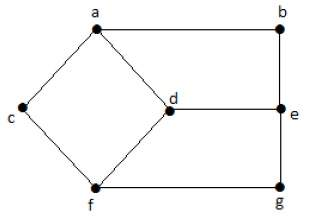# Distance between Vertices and Eccentricity

## Distance between Two Vertices

It is number of edges in a shortest path between Vertex U and Vertex V. If there are multiple paths connecting two vertices, then the shortest path is considered as the distance between the two vertices.

Notation − d(U,V)

There can be any number of paths present from one vertex to other. Among those, you need to choose only the shortest one.

## Example

Take a look at the following graph −Here, the distance from vertex 'd' to vertex 'e' or simply 'de' is 1 as there is one edge between them. There are many paths from vertex 'd' to vertex 'e' −

• da, ab, be
• df, fg, ge
• de (It is considered for distance between the vertices)
• df, fc, ca, ab, be
• da, ac, cf, fg, ge

## Eccentricity of a Vertex

The maximum distance between a vertex to all other vertices is considered as the eccentricity of vertex.

Notation − e(V)

The distance from a particular vertex to all other vertices in the graph is taken and among those distances, the eccentricity is the highest of distances.

## Example

In the above graph, the eccentricity of 'a' is 3.

The distance from 'a' to 'b' is 1 ('ab'),

from 'a' to 'c' is 1 ('ac'),

from 'a' to 'd' is 1 ('ad'),

from 'a' to 'e' is 2 ('ab'-'be') or ('ad'-'de'),

from 'a' to 'f' is 2 ('ac'-'cf') or ('ad'-'df'),

from 'a' to 'g' is 3 ('ac'-'cf'-'fg') or ('ad'-'df'-'fg').

So the eccentricity is 3, which is a maximum from vertex 'a' from the distance between 'ag' which is maximum.

In other words,

e(b) = 3

e(c) = 3

e(d) = 2

e(e) = 3

e(f) = 3

e(g) = 3

## Radius of a Connected Graph

The minimum eccentricity from all the vertices is considered as the radius of the Graph G. The minimum among all the maximum distances between a vertex to all other vertices is considered as the radius of the Graph G.

Notation − r(G)

From all the eccentricities of the vertices in a graph, the radius of the connected graph is the minimum of all those eccentricities.

Example − In the above graph r(G) = 2, which is the minimum eccentricity for 'd'.

## Diameter of a Graph

The maximum eccentricity from all the vertices is considered as the diameter of the Graph G. The maximum among all the distances between a vertex to all other vertices is considered as the diameter of the Graph G.

Notation − d(G)

From all the eccentricities of the vertices in a graph, the diameter of the connected graph is the maximum of all those eccentricities.

Example − In the above graph, d(G) = 3; which is the maximum eccentricity.